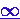Formulas used in Queueing theory

Note:
If You find that tables are too small to read, click them to enlarge.

Sections:

 1  Notation and definitions 2  Relationships between random variables 3  M/M/1 Formulas 4  M/M/1/K Formulas 5  M/M/c Formulas 6  M/M/2 Formulas 7  M/M/c/c Formulas 8  M/M/c/K Formulas 9  M/M/Formulas 10  M/M/1/K/K Formulas 11  M/G/1/K/K Formulas 12  M/M/c/K/K Formulas 13  D/D/c/K/K Formulas 14  M/G/1 Formulas 15  GI/M/1 Formulas 16  GI/M/c Formulas 17  M/G/1 Priority queueing system 18  M/M/c Priority queueing system